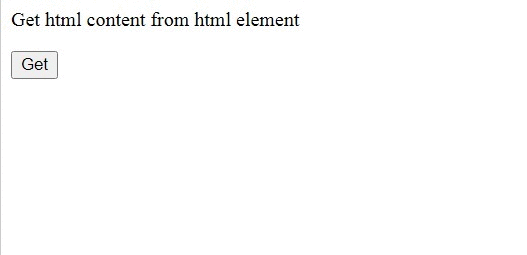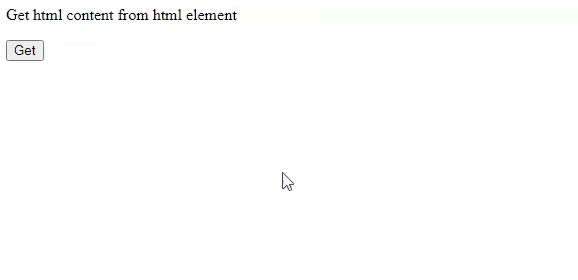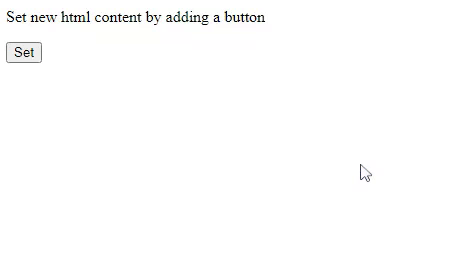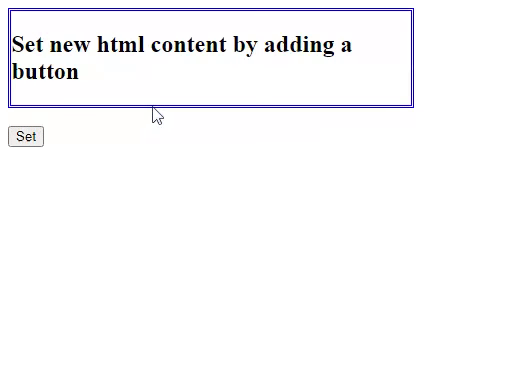GeeksforGeeks App
Open AppBrowser
Continue

# What is the use of html() method in jQuery ?

The html() method in jQuery is used to get the contents of the first element in the set of matched elements or is used to set the HTML contents of every matched element. It returns the content of the first matched element. This function does not accept any arguments.

Syntax:

`\$(selector).html();`

Approach: We will create a button with id & set its value as get. Then we write the jQuery script as alerting a simple message which returns the HTML contents of the first matched element once the user clicks the “Get” button.

Example: In this example, we are getting the contents of the <div> tag once the user clicks the button that will be displayed using the alert method.

## HTML

 ```<``html``>`` ` `<``head``>``    ``<``script` `type``=``"text/javascript"` `src``=``"https://code.jquery.com/jquery-1.12.0.min.js"``>``    ````     ` `    ``<``script``>``        ``\$(document).ready(function () {``            ``\$("button").click(function () {``                ``alert(\$("div").html());``            ``});``        ``});``    `````` ` `<``body``>``    ``<``div``>``         ` `<``p``>Get html content from html element`` ` `    ````    ``<``button` `id``=``"get"``>Get```` ` ``

Output:Converting the HTML element to a text:

Approach: We are creating a button with the value Get. Then we write the jQuery script that converts the contents of the HTML (which is the firstDiv element) into a string and displaying it in the paragraph element. We get the complete HTML contents of the first Div element in this example.

Example: In this example, we are getting the content of the div element. We have two div tags and only the contents of the first matched element will be returned.

## HTML

 ```<``html``>`` ` `<``head``>``    ``<``script` `type``=``"text/javascript"` `src``=``"https://code.jquery.com/jquery-1.12.0.min.js"``>``    ````     ` `    ``<``script``>``        ``\$(document).ready(function () {``            ``\$("button").click(function () {``                ``var str = \$("div.firstDiv").html();``                ``\$("p").text(str);``            ``});``        ``});``    `````` ` `<``body``>``    ``<``div` `class``=``"firstDiv"``>``        ``<``div` `class``=``"secondDiv"``>``             ` `<``p``>Get html content from html element`` ` `        ````    ````    ``<``button` `id``=``"get"``>Get```` ` ``

Output:Setting the contents of HTML:

Syntax:

It sets the content of the matched element.

`\$(selector).html(content)`

It sets the content using a function.

`\$(selector).html(function(index, currentcontent))`

Parameters: This method accepts two parameters as mentioned above and described below:

• content: It is a mandatory parameter that specifies the new content for the selected elements.
• function(index, currentcontent): It is an optional parameter that specifies a function that returns the new content for the selected element.
• index: It is used to return the index position of the element in the set.
• currentcontent: It is used to return the current HTML content of the selected element.

Approach: We are creating a button with the Value Set. Then we write the jQuery script that sets the contents of the first matched element i.e. the first Div element. The complete code of fisrtDiv i.e. a first Div element will be changed to “New HTML Content and GeeksforGeeks”. Note that we are using the <h1> and <h2> tags in the updated content of the HTML.

Example: In this example, we are setting the content of the div element once the user clicks the button Set using the first syntax.

## HTML

 ```<``html``>`` ` `<``head``>``    ``<``script` `type``=``"text/javascript"` `src``=``"https://code.jquery.com/jquery-1.12.0.min.js"``>``    ```` ` `    ``<``script``>``        ``\$(document).ready(function () {``            ``\$("button").click(function () {``                ``\$("div").html(``"<``h1``>New HTML Content <``h2``>GeeksforGeeks");``            ``});``        ``});``    `````` ` `<``body``>``    ``<``div` `class``=``"firstDiv"``>``        ``<``div` `class``=``"secondDiv"``>``             ` `<``p``>Set new html content by adding a button`` ` `        ````    ````    ``<``button``>Set```` ` ``

Output:Approach: We are creating a button with the Value Set. Then we write the jQuery script which sets the contents of the first matched element i.e., the first Div element using a function. The complete code of fisrtDiv i.e., a first Div element will be changed to “Old content is: Set new HTML content by adding a button with index 0 is now changed”. The function has two arguments i.e., index value and the old content as a string. We are using these values and updating the old content as you can see in the output.

Example: In this example, we are setting the contents of the first Div element once the user clicks on the button Set. We are simply returning a message and also printing the index value received.

## HTML

 ```<``html``>`` ` `<``head``>``    ``<``script` `type``=``"text/javascript"` `src``=``"https://code.jquery.com/jquery-1.12.0.min.js"``>``    ```` ` `    ``<``style``>``        ``.firstDiv {``            ``width: 400px;``            ``border-color: blue;``            ``border-width: 0.2em;``            ``border-style: double;``        ``}``    ```` ` `    ``<``script``>``        ``\$(document).ready(function () {``            ``\$("button").click(function () {``                ``\$("div").html(function (i, str) {``                    ``return (``                        ``"<``h2``>Old content is:" +``                        ``str +``                        ``"<``h2``>with index" +``                        ``i +``                        ``"<``h2``>is now changed"``                    ``);``                ``});``            ``});``        ``});``    `````` ` `<``body``>``    ``<``div` `class``=``"firstDiv"``>``        ``<``div` `class``=``"secondDiv"``>``            ``<``h2``>``                ``Set new html content ``                ``by adding a button``            ````        ````    ````    ``<``br` `/>``    ``<``button``>Set```` ` ``

Output:My Personal Notes arrow_drop_up
Related Tutorials